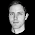## 2012-01-25

### Type level integers with Type Families (numtype-tf 0.1)

Pretty much since they were introduced into GHC I’ve been meaning to try to port the dimensional library to use Type Families instead of Functional Dependencies. However, functional dependencies have served me quite well and I’ve been lacking the inspiration to dig into type families. That is, until I read the Leonidas’ Statically Typed Vector Algebra Using Type Families. Leonidas’ blog post resonated with me as the problem it addresses is related to one I myself have been interested in for some time (linear algebra with static guarantees). It also gave me a clear vision of how to apply type families to the fundamendal problem of dimensional – type level integers.

I’ve implemented the numtype-tf library which is in essence numtype using type families (hence the “-tf”) instead of functional dependencies. Some of my design decisions are slightly different (beside the choice of type system extension), e.g. the representation of negative integers is different and the `PosType` and `NegType` classes have been dropped in numtype-tf. The haddocks have also been improved a little.

A feature of the functionally dependent numtype that I was unable to reproduce with type families is ”mutual dependencies”, used in the `Sum` and `Div` type classes of numtype. Here is an example of type signatures (instances omitted) and ghci usage:

``> class Sum a b c | a b -> c, a c -> b, b c -> a> (+) :: (Sum a b c) => a -> b -> c> (-) :: (Sum a b c) => c -> b -> a``
``````\*Numeric.NumType> pos3 - pos5
NumType -2
``````

To the best of my knowledge corresponding code with type families would be along the lines of:

``> type family Sum a b> (+) :: a -> b -> Sum a b> (-) :: Sum a b -> b -> a``
``````\*Numeric.NumType.TF> pos3 - pos5

<interactive>:1:1:
Couldn't match type `Sum a0 Pos5' with `S Pos2'
…
``````

In order for the latter to compute we must assist the type checker by being explicit about the type of `a0`, i.e. `pos3 - pos5 :: Neg2`, which largely defeats the purpose of type level arithmetic. Instead two type families are used in numtype-tf:

``> type family Add a b  -- a + b> (+) :: a -> b -> Add a b> type family Sub a b  -- a - b.> (-) :: a -> b -> Sub a b``

This works OK but the lack och mutual dependencies in type families restricts what I will losely refer to as “type inference back tracking”. This may or may not be a significant drawback compared to fundep numtype depending on the use case.

If you are interested in comparing code written with type families to code written with functional dependencies take a look at the respective modules on Github: Numeric.NumType.TF vs. Numeric.NumType.

The next step is to reimplement dimensional as dimensional-tf in order to exercise numtype-tf and better understand the impact of the differences with respect to numtype. I am also looking forward to playing with GHC.TypeNats in GHC 7.4.#### 2 comments:

1.You should be able to write something like this in recent GHC:

class (Add a b ~ c, Sub c a ~ b, Sub c b ~ a) => Sum a b c where
type Add a b
type Sub c a

This should give you back “type inference back tracking”, if I understand what you mean by that.

(Making Sum definitionally commutative by including both Sub c a ~ b and Sub c b ~ a might be too ambitious, though—you might have to discard the latter, or split subtraction into left and right subtraction.)

2.Thanks Andreas for pointing me in the right direction. On GHC 7.2.1 I seem to be able to write:

> class (Add a b ~ c, Add b a ~ c, Sub c a ~ b, Sub c b ~ a) => Sum a b c
> instance (Add a b ~ c, Add b a ~ c, Sub c a ~ b, Sub c b ~ a) => Sum a b c
> (+) :: Sum a b c => a -> b -> c
> (-) :: Sum a b c => c -> b -> a

Interestingly the type signatures for (+) and (-) are the same as the original numtype ones.